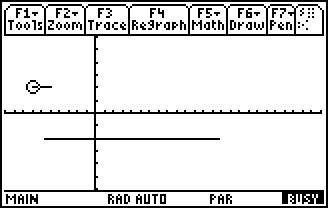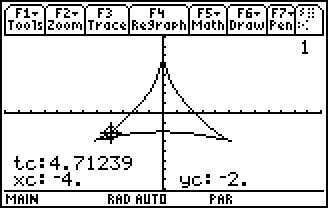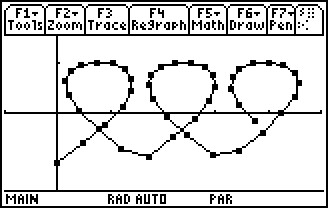# Activities

••• ##### Subject Area

• Math: Calculus: Parametric Equations and Polar Coordinates

• ##### Author9-12

20 Minutes

• ##### Device
• TI-89 / TI-89 Titanium

TI Connect™

• ##### Accessories

TI Connectivity Cable

## Parametric Races & Slopes#### Activity Overview

Students will study the motion of two points and use calculus to determine the velocity and acceleration. The slope of parametric equations will be determined.

#### Key Steps

•Students will investigate parametric equations. Students will study the motion of two points and use calculus to determine the velocity and acceleration.

•Next, students will explore a graph that is not a function, but can be graphed with parametric equations. They will explore what values of t result in cusps, horizontal and vertical tangents, and tangents equal to 1.

•As an extension, students will try to create a graph from parametric equations that matches some given points. Students will find the value(s) of t for the vertical and horizontal tangents.